# Multiplication and Division, Part 1

## Objective

Identify and create situations involving equal groups and describe these situations using the language and notation of multiplication.

## Common Core Standards

### Core Standards

?

• 3.OA.A.1 — Interpret products of whole numbers, e.g., interpret 5 × 7 as the total number of objects in 5 groups of 7 objects each. For example, describe a context in which a total number of objects can be expressed as 5 × 7.

?

• 2.NBT.A.2

• 2.OA.C.3

• 2.OA.C.4

## Criteria for Success

?

1. Understand that equal groups are groups made up of equal numbers of objects in each group.
2. Use the multiplication symbol to represent equal groups (MP.2).
3. Understand that multiplication cannot be used to represent situations with unequal groups.
4. Understand that the way we write a multiplication sentence is a convention where the first factor represents the number of groups and the second factor represents the size of the groups (MP.6).
5. Identify the number of groups, the size of each group, and the total number of objects in a context or picture of equal groups.
6. Understand that a factor is the number of groups or size of each group in a multiplication sentence and the product is the total number of objects in a multiplication sentence.

## Tips for Teachers

?

• The following material is needed for today's lesson: Counters
• Students will learn to write factors in the conventional order, namely with the number of groups written as the first factor and the size of the groups as the second factor. But, since “some students bring th[e] interpretation of multiplication equations [where the meaning of the factors is switched] into the classroom, …it is useful to discuss the different interpretations and allow students to use whichever is used in their home” (OA Progression, p. 25), if it comes up. Thus, use the conventional way of writing factors when addressing the whole class, but allow individual students to write them in reverse order if they have experience doing so and they are able to explain the meaning of each factor. In Lesson 7, students will learn the commutative property, after which all students will be given much more free reign over the order in which they write their factors.
• In this lesson, finding the product is introduced in the context of writing equations, but efficient methods for finding the product are not discussed until Topics B and C. For now, because images are included with every problem, students can count all (Level 1 strategy) to find the total, or they may see that skip-counting or repeated addition (Level 2 strategies) can be used to find the total. Focus on the conceptual understanding of multiplication here, since they will have lots of time to discuss strategies in later lessons.
• Throughout this unit, it is recommended to give students daily practice with skip-counting to build toward fluency with up to 1-digit by 1-digit multiplication. Below are some possible routines you can use with students. (Source for these routines: Jessica Shumway, Number Sense Routines: Building Numerical Literacy Every Day in Grades K–3, pp. 55–67, 2011)
• Choral Counting: Choral counting is simply skip-counting out loud as a whole class. It’s a good routine to use when learning new skip-counting sequences or when you notice a large number of students struggling with a particular skip-counting sequence. You could use a visual to help students with their counting, especially in the beginning, such as a hundreds chart or number line. This also helps students notice patterns in the count sequence. You can involve movement, as well, by having students count on their fingers, do jumping jacks with every count, etc. It might be difficult to catch incorrect counts from students, which could reinforce incorrect counts, so it’s recommended not to rely just on this routine. You can ask some basic questions about the count sequence, but because this routine really just helps introduce students to new sequences or ones that they struggle with, more rigorous questions can be saved for the other routines (which are in bullets below).
• Count around the Circle: Have one child start with the first number in the skip-counting sequence, and go around the circle having each child say one number. You could decide to write the numbers on the board as students say them, either as a scaffold for them or to encourage a discussion of patterns afterward. Some questions you can ask before/during/after this routine to encourage sense-making include:
• If we count by twos around the circle starting with Student A, what number do you think Student Z will say? If you didn’t count to figure that out, how did you solve?
• If we count around the circle by fives and we go around twice, what will Student X say?
• Why did you choose ____ as an estimate?
• Why didn’t anyone choose ____ as an estimate?
• How did you know what comes next?
• (After a child gets stuck but figures it out): What did you do to figure it out?
• (If you’ve written the numbers on the board as students counted): What do you notice? What do you wonder?
• As a supplement to the Problem Set, you can teach students the game Circles and Stars from YouCubed.com. (Since students will only work with factors 2–5 and 10 in this unit, you could either block out the 1 on the dice, tell them to roll again if they encounter that factor, or tell them to treat 1 as 10. Similarly, you’ll need to tell students what to do if they roll double sixes (although 6 with any factor besides itself and 1 is fine).)

#### Remote Learning Guidance

If you need to adapt or shorten this lesson for remote learning, we suggest prioritizing Anchor Tasks 1 and 2 (benefit from worked examples). Find more guidance on adapting our math curriculum for remote learning here.

#### Fishtank Plus

• Problem Set
• Student Handout Editor
• Vocabulary Package

?

### Problem 1

Are these equal groups? How do you know?

a.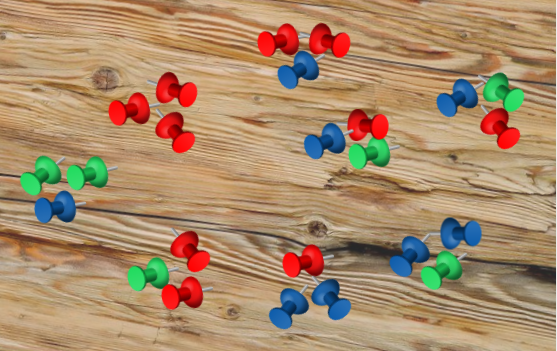b.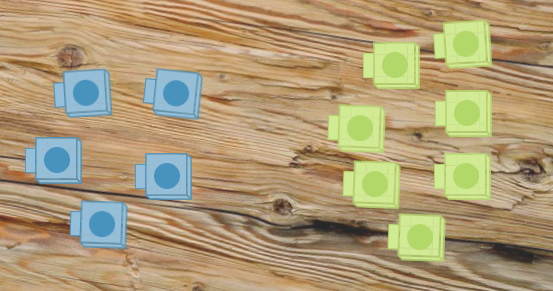### Problem 2

Instead of saying “8 groups of 3,” we’d write “$8\times3$,” which can we read out loud as “eight groups of three,” “eight times three,” or “eight multiplied by three.” We call this a multiplication expression.

a. Draw a model that represents 4 groups of 6. Then write a multiplication expression that matches your model.

The total number of objects in equal groups is called a product

b. What is the product in your model? Then write a multiplication equation that matches your model, including the product of it.

#### References

EngageNY Mathematics Grade 3 Mathematics > Module 1 > Topic A > Lesson 1Concept Development

Grade 3 Mathematics > Module 1 > Topic A > Lesson 1 of the New York State Common Core Mathematics Curriculum from EngageNY and Great Minds. © 2015 Great Minds. Licensed by EngageNY of the New York State Education Department under the CC BY-NC-SA 3.0 US license. Accessed Dec. 2, 2016, 5:15 p.m..

Modified by The Match Foundation, Inc.

### Problem 3

Write a multiplication sentence to represent the following picture. Make sure to write the factors in the conventional order.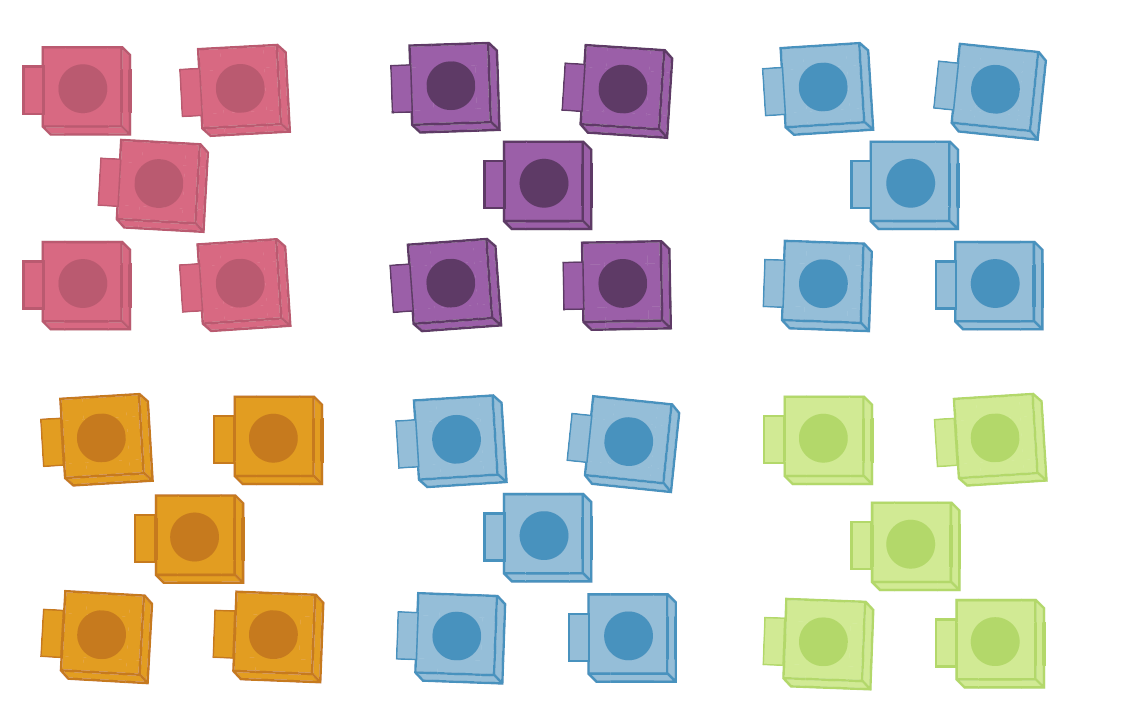## Problem Set & Homework

#### Discussion of Problem Set

• Identify the factors and their meanings from each image in #1.
• What did you notice about the answers to Parts (a) and (b) and Parts (c) and (d) in #1?
• Which models were correct in #5? How do you know? Which models were incorrect? Why?

?

For each image below,

• Determine whether it has equal groups. Explain how you know.
• If it does have equal groups, write a multiplication equation to represent it.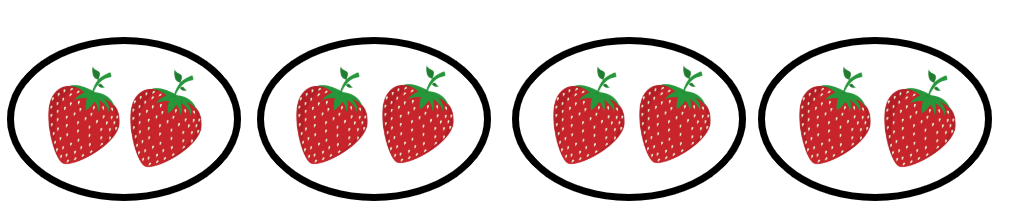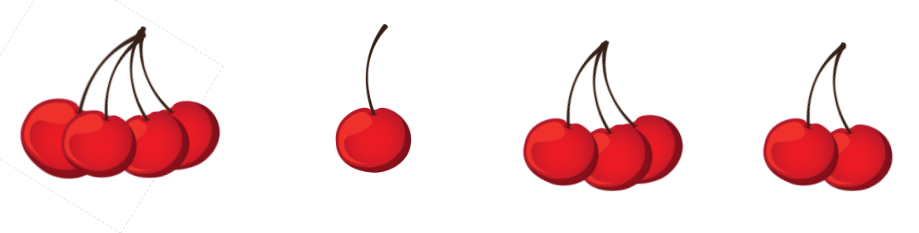### Mastery Response

?